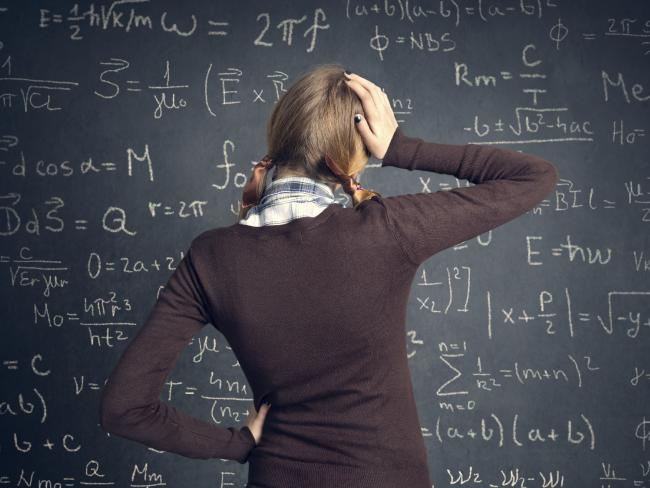This simple trick, which sees users able to work out their age and shoe size using a simple formula, is taking the internet by storm.

Basically, by taking your shoe size, multiplying it by 5, adding 50, multiplying that answer by 20, adding 1015 and then subtracting the year you were born, you should land on your shoe size and age.

Ahhh the magic of mathematics.

There are a few variables, in that the equation won’t work if you’ve already had your birthday in 2016, or you have a half shoe size (instead of 5, multiply your shoe size by 50 instead).

So, how does this fabulous formula actually work, and land on the correct shoe and age every time?

According to Professor Geoff Prince, director of the Australian Mathematical Sciences Institute, there’s sadly no real ‘magic’ in this mathematical trick.

“It’s actually a formula, and the 1015 is a real giveaway to how the answer is resolved,” Professor Prince told news.com.au.

“If you were to put the formula in to brackets, so (SHOE SIZE x 5 + 50) x 20 + 1015 — BIRTH YEAR, and break it down, you will actually get 100 x shoe size + age.

“If your birthday has already passed this year, change the 1015 to 1016 and you’ll get the age you’re turning in 2016, as well as your shoe size.”